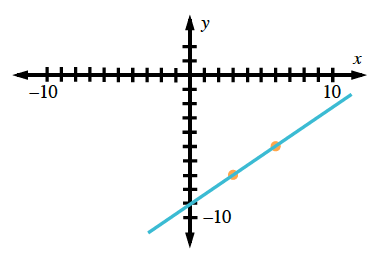### Home > A2C > Chapter 4 > Lesson 4.3.1 > Problem4-143

4-143.

The point $\left(3, −7\right)$ is on a line with a slope of $\frac { 2 } { 3 }$. Find another point on the line.

Plot the point $\left(3, −7\right)$, then count up and over the appropriate number of steps and place another point.Where is this new point located on the graph?

There is more than one correct answer.